The bond having the highest bond energy is :

(a) C=C

(b) C=S

(c) C=O

(d) P=N

High Yielding Test Series + Question Bank - NEET 2020

Difficulty Level:

H.O.M.O. (Highest Occupied Molecular Orbital) of CO molecular is :

(a) Non-bonding M.O. with slight antibonding character

(b) Non-bonding M.O. with slight bonding character

(c) Pure non-bonding M.O.

(d) None of the above

Concept Questions :-

V.S.E.P.R
High Yielding Test Series + Question Bank - NEET 2020

Difficulty Level:

Incorrect match is:

Electron geometry                                 Possible molecular shape from respective electron                                                                                                              geometery

(b) Trigonal bipyramidal                           -           Traingular planar

(c) Octahedron                                        -           Square Pyramidal

(d) Pentagonal bipyramidal                      -            Pentagonal planar

Concept Questions :-

Lattice/Hydration Energy
High Yielding Test Series + Question Bank - NEET 2020

Difficulty Level:

In which of the following species central atom is NOT surrounded by exactly 8 valence electrons?

(a) $B{{F}_{4}}^{-}$

(b) $NC{l}_{3}$

(c) $PC{{l}_{4}}^{+}$

(d) $S{F}_{4}$

High Yielding Test Series + Question Bank - NEET 2020

Difficulty Level:

The structure of ${\mathrm{B}}_{3}{\mathrm{N}}_{3}{\mathrm{H}}_{6}$ is as follows :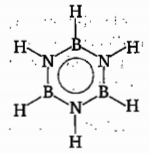How many derivative structures of ${\mathrm{B}}_{3}{\mathrm{N}}_{3}{\mathrm{H}}_{4}{\mathrm{X}}_{2}$ can be derived from the basic structure, by the

replacement of two hydrogen atoms?

(a) 2                                         (b) 3

(c) 4                                         (d) 5

Concept Questions :-

Hybridisation
High Yielding Test Series + Question Bank - NEET 2020

Difficulty Level:

Correctly match is :

(a) ${\mathrm{d}}_{{\mathrm{x}}^{2}-{\mathrm{y}}^{2}}$ atomic orbital                                    $—$One nodal plane

(b) ${P}_{y}$ atomic orbital                                          $—$Two nodal plane

(c) ${\sigma }_{px}$                                                              $—\psi$ (gerade)

(d) ${P}_{py}$                                                              $—\psi$ (ungerade)

Concept Questions :-

Covalent Bonding
High Yielding Test Series + Question Bank - NEET 2020

Difficulty Level:

In which of the following molecular shape ${d}_{{z}^{2-}}$ orbital must not be involved in bonding?

(a) Pentagonal planar

(b) Trigonal planar

(c) Linear

(d) Square planar

Concept Questions :-

Hybridisation
High Yielding Test Series + Question Bank - NEET 2020

Difficulty Level:

Consider the following reaction:

${\mathrm{MX}}_{4}+\mathrm{X}{\text{'}}_{2}\to {\mathrm{MX}}_{4}\mathrm{X}{\text{'}}_{2}$

If atomic number of M is 52 and X and X' are halogens and X' is more electronegative than X.

Then choose correct statement regarding given information:

(a) Both X' atoms occupy axial positions which are formed by overlapping of p and d-orbitals only

(b) All

(c) Central atom 'M' does not use anyone valence non-axial set of d-orbital in hybridization of final product

(d) Hybridization of central atom 'M' remains same in both reactant and final product.

Concept Questions :-

Hybridisation
High Yielding Test Series + Question Bank - NEET 2020

Difficulty Level:

Which atom can have more than eight valence electrons when it is forming covalent bond?

(a) H

(b) N

(c) F

(d) Cl

Concept Questions :-

Types of Bonding
High Yielding Test Series + Question Bank - NEET 2020

Difficulty Level:

Select the incorrect match:

Statement                               Shape                                    Example

(a) Bond pair has > 75%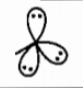HF

p-character

(b) Reduction in axial bond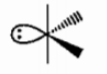${\mathrm{SF}}_{4}$

is more than that of in

equatorial bond angle

(c) Two axial d-orbitals and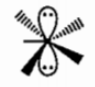${\mathrm{XeF}}_{5}^{-}$

one non-axial d-orbitals are

used in hybridization

(d) Two p-orbitals are used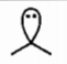${\mathrm{SnCl}}_{2}$

in hybridization

Concept Questions :-

Hybridisation